## A baseball of mass m1 = 0.35 kg is thrown at another ball hanging from the ceiling by a length of string L = 1.35 m. The second ball m2 = 0.

Question

A baseball of mass m1 = 0.35 kg is thrown at another ball hanging from the ceiling by a length of string L = 1.35 m. The second ball m2 = 0.93 kg is initially at rest while the baseball has an initial horizontal velocity of V1 = 3.5 m/s. After the collision the first baseball falls straight down (no horizontal velocity).

What is the angle that the string makes with the vertical at the highest point of travel in degrees?

in progress 0
6 months 2021-08-04T19:51:00+00:00 1 Answers 45 views 0

the approximately angle = 34⁰

Explanation:

given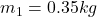length of string  L = 1.35 m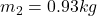initial horizontal velocity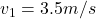suppose ball will rise up to a height h as

h = L – L cos θ

h = 1.35 – 1.35  cos θ

1.05 cos θ = 1.35 – h

at the maximum height,  we use conservation of kinetic and potential energy. As the 2nd ball rises to its maximum height, its kinetic energy  will convert  into potential energy.

KE = PE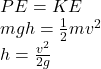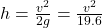we kinetic energy of the second ball after the collision. To determine the 2nd ball velocity after the collision,

use conservation of momentum.

Initial momentum of 1st ball = 0.35 × 3.5 = 1.225   ……….(i)

Since the baseball has no horizontal velocity after the collision, all of its momentum is transferred to the 2nd ball.

momentum for second ball  = mv = 0.93 v ……(ii)

equate (i) and  (ii) we get

0.93 v = 1.225

v = 1.32 m/s  ………(iii)

use this value to find height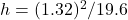This is approximately 0.088 meter

cos θ = (1 – 0.08)/1.35

cos θ = 0.9407

the approximately angle = 34⁰